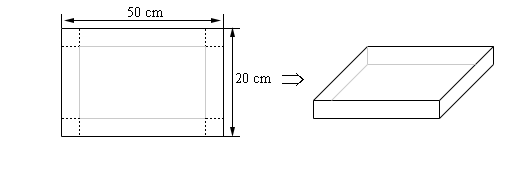Paul's Online Notes
Home / Calculus I / Applications of Derivatives / Optimization
Show Mobile Notice Show All Notes Hide All Notes
Mobile Notice
You appear to be on a device with a "narrow" screen width (i.e. you are probably on a mobile phone). Due to the nature of the mathematics on this site it is best views in landscape mode. If your device is not in landscape mode many of the equations will run off the side of your device (should be able to scroll to see them) and some of the menu items will be cut off due to the narrow screen width.

### Section 4-8 : Optimization

1. Find two positive numbers whose sum is 300 and whose product is a maximum. Solution
2. Find two positive numbers whose product is 750 and for which the sum of one and 10 times the other is a minimum. Solution
3. Let $$x$$ and $$y$$ be two positive numbers such that $$x + 2y = 50$$ and $$\left( {x + 1} \right)\left( {y + 2} \right)$$ is a maximum. Solution
4. We are going to fence in a rectangular field. If we look at the field from above the cost of the vertical sides are $10/ft, the cost of the bottom is$2/ft and the cost of the top is $7/ft. If we have$700 determine the dimensions of the field that will maximize the enclosed area. Solution
5. We have 45 m2 of material to build a box with a square base and no top. Determine the dimensions of the box that will maximize the enclosed volume. Solution
6. We want to build a box whose base length is 6 times the base width and the box will enclose 20 in3. The cost of the material of the sides is $3/in2 and the cost of the top and bottom is$15/in2. Determine the dimensions of the box that will minimize the cost. Solution
7. We want to construct a cylindrical can with a bottom but no top that will have a volume of 30 cm3. Determine the dimensions of the can that will minimize the amount of material needed to construct the can. Solution
8. We have a piece of cardboard that is 50 cm by 20 cm and we are going to cut out the corners and fold up the sides to form a box. Determine the height of the box that will give a maximum volume.Solution